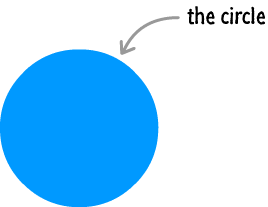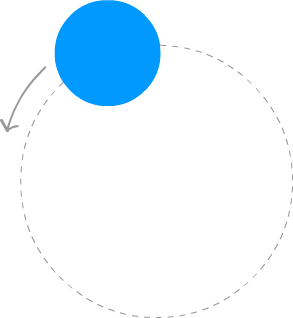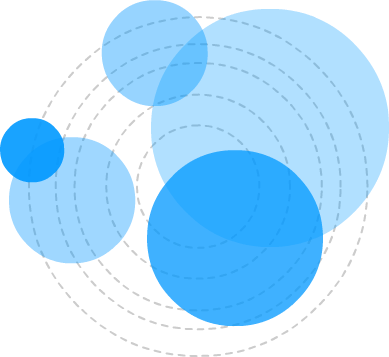﻿ Amorphous Circles | kirupa.com

Color

Background

Done

# Amorphous Circles

by kirupa   |   14 June 2013

A while ago, I was trying to create an animation that had circles oscillating around a fixed axis. Along the way towards getting that working, I ended up creating something a little bit different. That different something is what I call Amorphous Circles, and you can see it below.

Exactly fifty circles that are individually barely visible run over each other to create the blob-like effect that you see. This animation is a great example of what happens when you accidentally let some code run wild in your browser, but even accidents can teach you something valuable. In this short tutorial, let's quickly look at the various tricks involved in creating this animated effect.

This tutorial uses tips and techniques that I've covered extensively in other tutorials. To make the most of what is going on, I highly recommend you take a few moments and be familiar with the following content: Animating Many Things on a Canvas, Introduction to Easing in JavaScript, Creating a Simple HTML5 Canvas Animation, Animating in Code Using JavaScript.

I won't deny it. Yes, this is like a lot of pre-requisite reading if you want to fully understand what is going on.

Onwards!

## Overview of How It Works

Before looking at the code, let's look at how this effect works without getting too technical. For simplicity, I am going to focus on just one circle initially. Here is our circle:The way this effect works is by making a lot of circles move in a circular path. Our circle is not immune to this task:If you look closely at the overall animation, you only see a slight glimpse of this kind of movement. The reason is that, while a single circle moving circularly is pretty boring, things get pretty interesting when you get a lot of circles moving in a circular path. To make this ensuing chaos not look horrible, each of these circles has a different size, movement speed, and rotation radius.

Here is what our example looks like with just five circles thrown into the mix:With just five circles, you can see how this effect is starting to come together. Each circle is moving at its own pace and showing off its own unique visual properties. Once you have fifty of these circles, you get something that looks similar to the effect that you can see right now:This looks kind of messy, but that is because a static picture of this effect is a weak substitute for the real thing. In the next section, let's look at how the actual effect with all of its animation-ness works.

## Looking at the Code

Now that you've already seen how this effect works, the code is reasonably straightforward. In a nutshell, your code draws each circle, updates each circle's position, clears the screen, and starts all over again. This sounds pretty simple, and it is despite what may seem like a large amount of code.

The code for this entire effect is as follows:

```var mainCanvas = document.getElementById("myCanvas");
var mainContext = mainCanvas.getContext('2d');

var canvasWidth = mainCanvas.width;
var canvasHeight = mainCanvas.height;

// the cornerstone to any nutritious animation
var requestAnimationFrame = window.requestAnimationFrame ||
window.mozRequestAnimationFrame ||
window.webkitRequestAnimationFrame ||
window.msRequestAnimationFrame;

// this array contains a reference to every circle that you will create
var circles = new Array();

//
// The Circle "constructor" is responsible for creating the circle
// objects and defining the various properties they have
//
this.angle = angle;
this.sign = sign;
this.initialX = initialX;
this.initialY = initialY;
this.incrementer = .01 + Math.random() * .1;
}

Circle.prototype.update = function () {

this.angle += this.incrementer;

this.currentX = this.initialX + this.rotationRadius * Math.cos(this.angle);
this.currentY = this.initialY + this.rotationRadius * Math.sin(this.angle);

if (this.angle >= (Math.PI * 2)) {
this.angle = 0;
this.incrementer = .01 + Math.random() * .1;
}

// The following code is responsible for actually drawing the circle
mainContext.beginPath();
0, Math.PI * 2, false);
mainContext.closePath();
mainContext.fillStyle = 'rgba(0, 51, 204, .1)';
mainContext.fill();
};

//
// This function creates the circles that you end up seeing
//
function createCircles() {
// change the range of this loop to adjust the number of circles you see
for (var i = 0; i < 50; i++) {
var radius = 5 + Math.random() * 100;
var initialX = canvasWidth / 2;
var initialY = canvasHeight / 2;
var rotationRadius = 1 + Math.random() * 30;
var angle = Math.random() * 2 * Math.PI;

var signHelper = Math.floor(Math.random() * 2);
var sign;

// Randomly specify the direction the circle will be rotating
if (signHelper == 1) {
sign = -1;
} else {
sign = 1;
}

// create the Circle object
var circle = new Circle(angle,
sign,
initialX,
initialY);
circles.push(circle);
}
// call the draw function approximately 60 times a second
draw();
}
createCircles();

function draw() {
mainContext.clearRect(0, 0, canvasWidth, canvasHeight);
mainContext.fillStyle = '#F6F6F6';
mainContext.fillRect(0, 0, canvasWidth, canvasHeight);

for (var i = 0; i < circles.length; i++) {
var circle = circles[i];
circle.update();
}

// call the draw function again!
requestAnimationFrame(draw);
}```

Take a few moments to look through this code. If you followed some of the recommended pre-requisite tutorials I listed at the beginning, everything here should look familiar. If things don't look familiar, that's OK was well. We'll quickly walk through the code in the following sections.

### Creating our Circles

The first function that gets called is createCircles:

```function createCircles() {
// change the range of this loop to adjust the number of circles you see
for (var i = 0; i < 50; i++) {
var radius = 5 + Math.random() * 100;
var initialX = canvasWidth / 2;
var initialY = canvasHeight / 2;
var rotationRadius = 1 + Math.random() * 30;
var angle = Math.random() * 2 * Math.PI;

var signHelper = Math.floor(Math.random() * 2);
var sign;

// Randomly specify the direction the circle will be rotating
if (signHelper == 1) {
sign = -1;
} else {
sign = 1;
}

// create the Circle object
var circle = new Circle(angle,
sign,
initialX,
initialY);
circles.push(circle);
}
// call the draw function approximately 60 times a second
draw();
}```

As its name implies, this function's job is to create the Circle objects that ultimately result in each circle that you see drawn on the screen. You can see all of the properties that we define - properties that end up affecting how each circle looks or moves.

All of these relevant properties are passed in to the Circle constructor with the resulting object getting added to our circles array:

```var circle = new Circle(angle,
sign,
initialX,
initialY);
circles.push(circle);```

Once this for loop has run to completion, what you have left is 50 Circle objects that each can be accessed via our circles array. Keep the memory of this array on your fingertips. You'll run into it a few more times.

### The Circle Object

As you just saw, in the createCircles function, we create the Circle objects. What we haven't looked at is what exactly a Circle object is? Well...it is the following:

```function Circle(angle, sign, radius, rotationRadius, initialX, initialY) {
this.angle = angle;
this.sign = sign;
this.initialX = initialX;
this.initialY = initialY;
this.incrementer = .01 + Math.random() * .1;
}```

This Circle constructor that creates our Circle objects is nothing more than a collection of properties whose values are what gets passed in. These lines of code ensure each Circle object contains a property and value for angle, sign, radius, rotationRadius, initialX, initialY, and incrementer.

There is nothing particularly exciting going on here. The exciting part is what happens in our Circle object's update prototype:

```Circle.prototype.update = function () {

this.angle += this.incrementer;

this.currentX = this.initialX + this.rotationRadius * Math.cos(this.angle);
this.currentY = this.initialY + this.rotationRadius * Math.sin(this.angle);

if (this.angle >= (Math.PI * 2)) {
this.angle = 0;
this.incrementer = .01 + Math.random() * .1;
}

// The following code is responsible for actually drawing the circle on the screen
mainContext.beginPath();
0, Math.PI * 2, false);
mainContext.closePath();
mainContext.fillStyle = 'rgba(0, 51, 204, .1)';
mainContext.fill();
};```

We haven't seen when this function gets called yet, but know that when this function does get called, epic things happen. First, your circle's position properties are updated:

```this.angle += this.incrementer;

this.currentX = this.initialX + this.rotationRadius * Math.cos(this.angle);
this.currentY = this.initialY + this.rotationRadius * Math.sin(this.angle);

if (this.angle >= (Math.PI * 2)) {
this.angle = 0;
this.incrementer = .01 + Math.random() * .1;
}```

Second, these updated position properties are used to actually draw your circle on the screen:

```mainContext.beginPath();
mainContext.arc(this.currentX, this.currentY, this.radius, 0, Math.PI * 2, false);
mainContext.closePath();
mainContext.fillStyle = 'rgba(0, 51, 204, .1)';
mainContext.fill();```

If what you see here looks a bit strange, read the Drawing Circles on a Canvas tutorial when you get a chance.

### Drawing our Circles

We created our Circle objects and we also looked at what exactly our Circle object does. All that is left is tying everything together and looking at our draw function:

```function draw() {
mainContext.clearRect(0, 0, canvasWidth, canvasHeight);
mainContext.fillStyle = '#F6F6F6';
mainContext.fillRect(0, 0, canvasWidth, canvasHeight);

for (var i = 0; i < circles.length; i++) {
var circle = circles[i];
circle.update();
}

// call the draw function again!
requestAnimationFrame(draw);
}```

The draw function is responsible for clearing your canvas, going through each Circle object in the circles array, and calling the update function on each Circle object. Because this function is also the target for your requestAnimationFrame function, this function gets called around 60 times a second.

## Conclusion

If there was ever a tutorial that touched upon so many different animation-in-code concepts, this one takes the cake. If you know almost everything about what every line of code in this animation does, consider yourself ahead of the game when it comes to understanding how animations in code work.

Just a final word before we wrap up. If you have a question and/or want to be part of a friendly, collaborative community of over 220k other developers like yourself, post on the forums for a quick response!